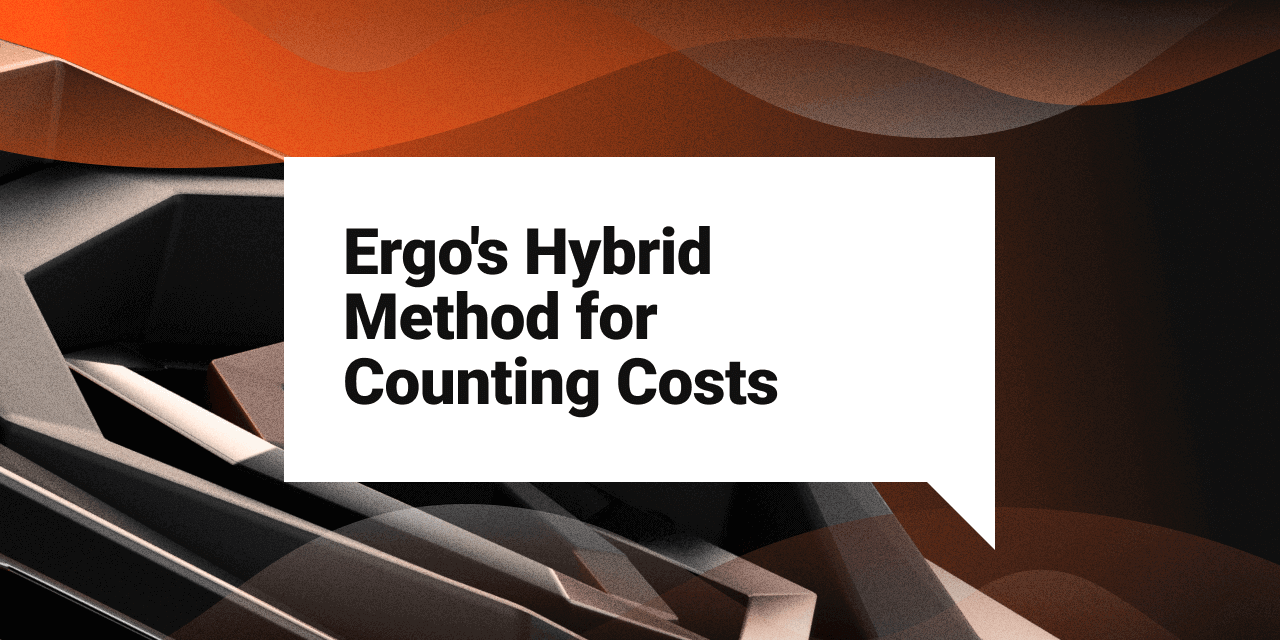# Ergo’s Hybrid Method for Counting Costs

Explainers
Ergo Platform

February 9, 2022## Introduction

Checking the validity of smart contracts on a Proof of Work (PoW) blockchain has costs, both in time and resources. If these costs are not controlled, it can lead to a slow network or even denial of service attacks that prevent the network from being used at all. Therefore, cost control is fundamental to a working blockchain.

Typically, there are two general ways to control costs on a PoW blockchain:

1. Ahead-Of-Time Costing (AOT Costing) - estimates costs before script execution so that an over-expensive script is not executed at all
2. Just-In-Time Costing (JIT Costing) - calculates costs during the actual execution of a script

Each of these methods has advantages and disadvantages. The upcoming v.5.0 upgrade of Ergo’s nodes uses a hybrid approach to improve cost control.

Currently, Ergo uses AOT costing. Work is done on the Ergo blockchain in blocks. Each block takes about 2 minutes and it includes all the transactions for that period of time. Blocks are given a budget and they cannot exceed that budget.

When a miner creates a block, the cost of the block is estimated ahead of time. If the cost is below the block’s budget and the math/logic adds up, the block is submitted to the blockchain.

However, estimates also have a cost. AOT costing makes sense when those costs are less than actually running the script. It is less expensive to estimate when using simple smart contract languages.

Using AOT costing has one main advantage. Limits are imposed by the network in blocks rather than by transaction. Since estimation costs are low, miners absorb transaction costs for transactions that are rejected. Each accepted transaction then requires a simple fee to be added to the block.

It is important to note that AOT costing is not without disadvantages. Estimates are not actual costs and are often inefficient, which wastes network resources, reduces each block’s capacity and by extension, the blockchain’s ability to scale. This is particularly true in cases such as Ergo, where the blockchain supports the use of collections of inputs and outputs, user-defined context extension variables, and other sophisticated features. Additionally, AOT costing does not take advantage of the efficiencies of next-generation smart contract languages that are less expensive to run than to estimate.

There are other disadvantages to AOT costing, but the above establishes the need for a better model of cost control than AOT costing alone.

## Ergo’s New Hybrid Method for Counting Costs

The upcoming Ergo node v.5.0 release will use a hybrid costing algorithm to contain costs and protect the network from denial-of-service, spam and other attacks on network resources. With this new approach, there are two stages to the costing algorithm.

In the first stage, transaction inputs go through a verifier (one input after another) and each input’s script is reduced to a sigma proposition - a kind of spending condition which can be cryptographically verified. JIT costing is applied during this reduction stage.

When every input in the transaction has a sigma proposition, then AOT costing (which is both very simple and fast) is used to calculate the costs of all cryptographic operations in the transaction. All costs are then added together to determine the final cost of the transaction itself. Once the final cost of the transaction is obtained it is added to the block cost, which must be within the block budget before it can be added to it.

The same process is repeated for each transaction in the block (in sequence) and the total cost of the block is accrued. Before it can be submitted to the blockchain, each block must be below the cost allowed per block.

With the deployment of this new two-part costing method, costs can be established more efficiently and accurately with less expense than using AOT costing alone. As a result, the release of Ergo node v.5.0 should effectively increase the number of transactions per block, improve cost estimates, and enhance the performance of the Ergo blockchain.

Share post